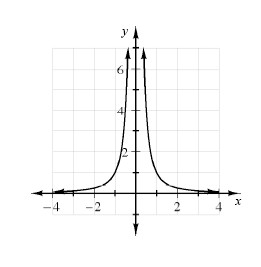### Home > A2C > Chapter 12 > Lesson 12.1.1 > Problem12-25

12-25.

The graph of $y = \frac { 1 } { x ^ { 2 } }$ is shown below.

1. What is the domain of the function?

Are there any vertical asymptotes or holes?

2. What is the range of the function?

Are there any horizontal asymptotes?

3. Solve $\frac { 1 } { x ^ { 2 } } = 4$

Use the graph.

4. Solve $\frac { 1 } { x ^ { 2 } } < 4$

Use the graph.